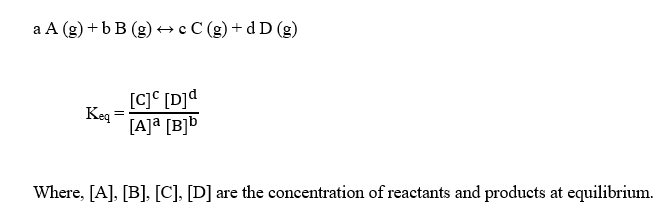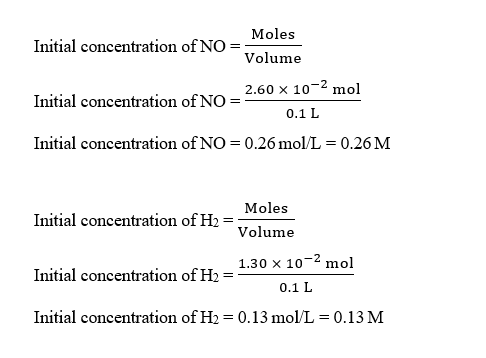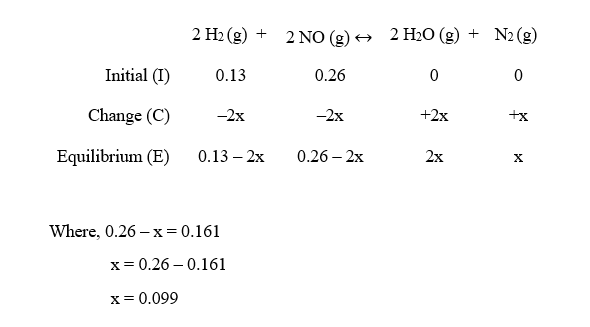# A 100.-mL reaction vessel initially contains 2.60 × 10–2 mol of NO and 1.30 × 10–2 mol of H2. At equilibrium, the concentration of NO in the vessel is 0.161 M. At equilibrium the vessel also contains N2, H2O, and H2.The balanced equation for the reaction is given below. 2H2​(g)+2NO(g)<------>2H2​O(g)+N2(g)Determine the equilibrium concentration of H2O (Water)

Question
87 views

A 100.-mL reaction vessel initially contains 2.60 × 10–2 mol of NO and 1.30 × 10–2 mol of H2. At equilibrium, the concentration of NO in the vessel is 0.161 M. At equilibrium the vessel also contains N2, H2O, and H2.

The balanced equation for the reaction is given below.

2H2​(g)+2NO(g)<------>2H2​O(g)+N2(g)

Determine the equilibrium concentration of H2O (Water)

check_circle

Step 1

The equilibrium constant, Keq for a reaction is given as :Step 2

Given,

Volume of vessel = 100 mL = 0.1 L   (1 mL = 0.001 L)

Initial moles of NO = 2.60 × 10-2 mol

Initial moles of H2 = 1.30 × 10-2 mol

Equilibrium concentration of NO = 0.161 M

Initial concentration of NO and H2 can be calculated as :Step 3

The balanced reaction is given as :

2 H2 (g) + 2 NO (g) ↔ 2 H2O (g) + N2 (g)

The ICE table for this reaction can be written as:...

### Want to see the full answer?

See Solution

#### Want to see this answer and more?

Solutions are written by subject experts who are available 24/7. Questions are typically answered within 1 hour.*

See Solution
*Response times may vary by subject and question.
Tagged in